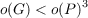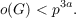#### Vol. 29, No. 2, 1969

 Download this articleFor screen For printingRecent Issues Vol. 325: 1  2 Vol. 324: 1  2 Vol. 323: 1  2 Vol. 322: 1  2 Vol. 321: 1  2 Vol. 320: 1  2 Vol. 319: 1  2 Vol. 318: 1  2Online Archive Volume: Issue:The Journal Subscriptions Editorial Board Officers Contacts Submission Guidelines Submission Form Policies for Authors ISSN: 1945-5844 (e-only) ISSN: 0030-8730 (print) Special Issues Author Index To Appear Other MSP Journals
On finite groups with independent cyclic Sylow subgroups

### Marcel Herzog

Vol. 29 (1969), No. 2, 285–293
##### Abstract

The purpose of this paper is to classify all perfect groups with cyclic Sylow p-subgroups which satisfy the condition

(TI) two different Sylow p-subgroups of G contain only the unit element in common and such thatwhere P is a Sylow p-subgroup of G.

The main result of this paper is the following

Theorem 1. Let G be a perfect finite group with a cyclic Sylow p-subgroup P of order pa and assume that the Sylow p-subgroups of G satisfy the (TI) condition. Assume, furthermore, thatThen one of the following statements holds.

(I) a = 1, GPSL(2,p), where p > 3 is a prime.

(II) a = 1, GPSL(2,p 1), where p = 2m + 1 > 5 is a Fermat prime.

(III) a = 1, GSL(2,p), where p > 3 is a prime.

(IV) a = 2, p = 3, GPSL(2,8).

Primary: 20.43
##### Milestones